## F.2.1 The Phonon GREEN's Function

The density-density correlation function of the nuclei is reduced in the following to a quantity of more practical interest, namely the phonon GREEN's function within the harmonic approximation. One can expand the ionic charge density up to first-order in the lattice displacement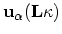with respect to the equilibrium positions of ions (see (F.25))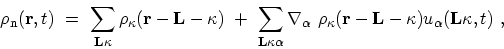(F.38)

where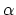denotes the Cartesian components. This expansion reduces the density-density correlation function (F.34) to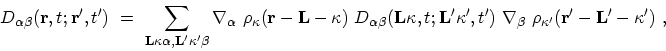(F.39)

where the phonon GREEN's function in real space is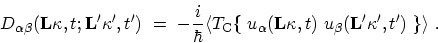(F.40)

Owing to the lattice periodicity of the ionic charge densities, the spatial FOURIER transformation of (F.39) takes the form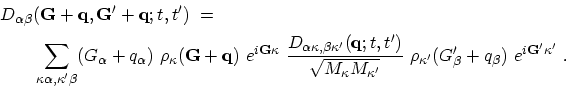(F.41)

The FOURIER expansion of the lattice displacement can be written as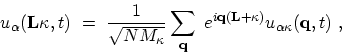(F.42)

where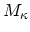are the mass of the atoms and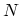is the number of atoms in the unit cell. By means of (F.42), the FOURIER transformation of (F.40) is given by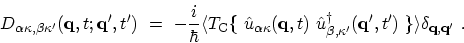(F.43)

By diagonalizing the dynamical matrix , one obtains the eigen-modes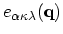and eigen-frequencies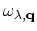of the lattice vibrations. These eigen-vectors can be used to expand the FOURIER components of the displacement in terms of phonon operators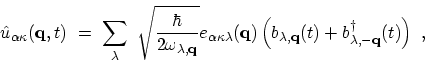(F.44)

where these operators have the time dependence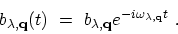(F.45)

This eigen-vector expansion allows one to factorize (F.43) for each phonon mode according to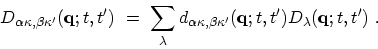(F.46)

into a weight factor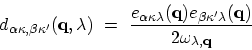(F.47)

and the phonon GREEN's function (Appendix D.2)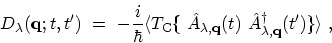(F.48)

where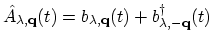,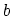, and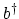are the annihilation and creation operators for Bosons. This factorization allows one to evaluate the coupling for any combination of phonon branch indices.

M. Pourfath: Numerical Study of Quantum Transport in Carbon Nanotube-Based Transistors